Next: Fourier Analysis Up: Longitudinal Standing Waves Previous: Longitudinal Waves on a

# Sound Waves in an Ideal Gas

Consider a uniform ideal gas of equilibrium mass density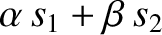and equilibrium pressure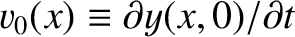. Let us investigate the longitudinal oscillations of such a gas. Such oscillations are known as sound waves. Generally speaking, a sound wave in an ideal gas oscillates sufficiently rapidly that heat is unable to flow fast enough to smooth out any temperature perturbations generated by the wave. Under these circumstances, the gas obeys the adiabatic gas law (Reif 2008),(323)

whereis the pressure,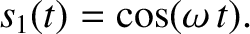the volume, and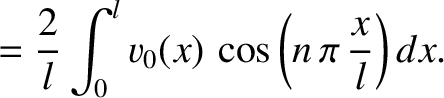the ratio of specific heats (i.e., the ratio of the gas's specific heat at constant pressure to its specific heat at constant volume) (ibid.). This ratio is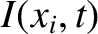for ordinary air (Haynes and Lide 2011b).

Consider a sound wave in a column of gas of cross-sectional area. Let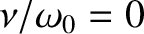measure distance along the column. Suppose that the wave generates an-directed displacement of the column,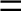. Consider a small section of the column lying between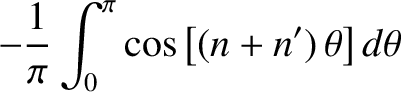and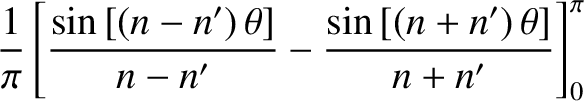. The change in volume of the section is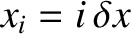. Hence, the relative change in volume, which is assumed to be small, is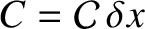(324)

In the limit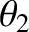, this becomes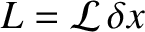(325)

The pressure perturbation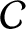associated with the volume perturbation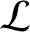follows from Equation (323), which yields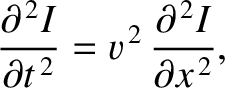(326)

or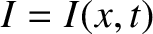(327)

giving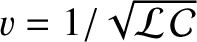(328)

where use has been made of Equation (325).

Consider a section of the gas column lying betweenand. The mass of this section is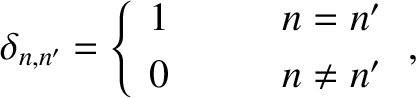. The-directed force acting on its left boundary is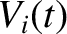, whereas the-directed force acting on its right boundary is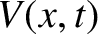. Finally, the average longitudinal (i.e.,-directed) acceleration of the section is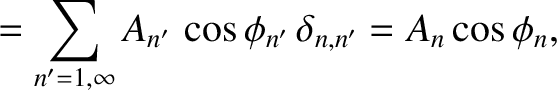. Thus, the section's longitudinal equation of motion is written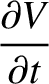(329)

In the limit, this equation reduces to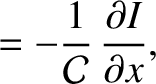(330)

Finally, Equation (328) yields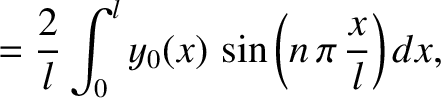(331)

where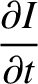(332)

is a constant with the dimensions of velocity, which turns out to be the sound speed in the gas. (See Section 7.1.)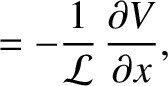As an example, suppose that a standing wave is excited in a uniform organ pipe of length. Let the closed end of the pipe lie at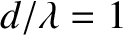, and the open end at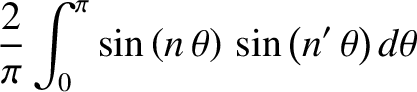. The standing wave satisfies the wave equation (331), whererepresents the speed of sound in air. The boundary conditions are that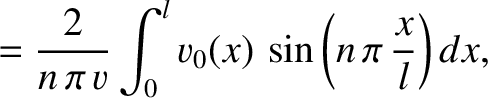--that is, there is zero longitudinal displacement of the air at the closed end of the pipe--and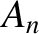--that is, there is zero pressure perturbation at the open end of the pipe (because the small pressure perturbation in the pipe is not intense enough to modify the pressure of the atmosphere external to the pipe). Let us write the displacement pattern associated with the standing wave in the form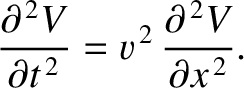(333)

where,,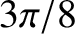, andare constants. This expression automatically satisfies the boundary condition. The other boundary condition is satisfied provided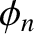(334)

which yields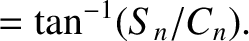(335)

where the mode number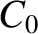is a positive integer. Equations (331) and (333) give the dispersion relation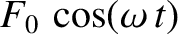(336)

Hence, theth normal mode has a wavelength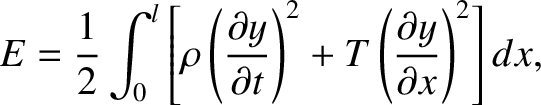(337)

and an oscillation frequency (in hertz)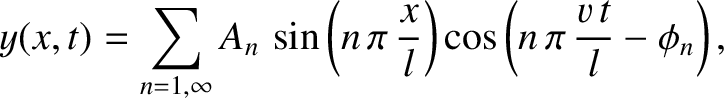(338)

where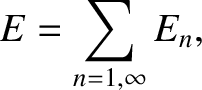is the frequency of the fundamental harmonic (i.e., the normal mode with the lowest oscillation frequency). Figure 34 illustrates (in a schematic fashion) the characteristic displacement patterns and oscillation frequencies of the pipe's first three normal modes (i.e.,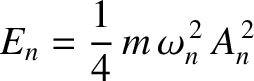, and 3). It can be seen that the modes all have a node at the closed end of the pipe, and an anti-node at the open end. The fundamental harmonic has a wavelength that is four times the length of the pipe. The first overtone harmonic has a wavelength that is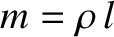rds the length of the pipe, and a frequency that is three times that of the fundamental. Finally, the second overtone has a wavelength that is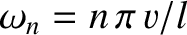ths the length of the pipe, and a frequency that is five times that of the fundamental. By contrast, the normal modes of a guitar string have nodes at either end of the string. (See Figure 24.) Thus, the fundamental harmonic has a wavelength that is twice the length of the string. The first overtone harmonic has a wavelength that is the length of the string, and a frequency that is twice that of the fundamental. Finally, the second overtone harmonic has a wavelength that is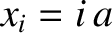rds the length of the string, and a frequency that is three times that of the fundamental.Next: Fourier Analysis Up: Longitudinal Standing Waves Previous: Longitudinal Waves on a
Richard Fitzpatrick 2013-04-08